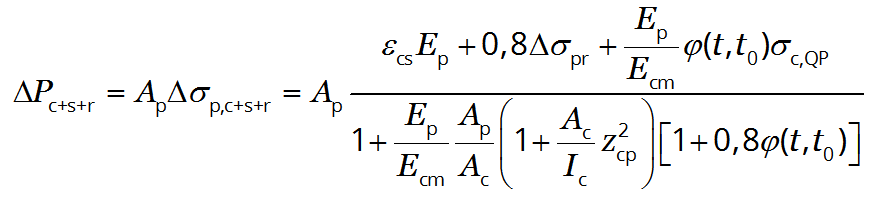For full functionality of this site it is necessary to enable JavaScript. Here are the instructions how to enable JavaScript in your web browser.

# Time dependent losses of prestress due to creep, shrinkage and relaxation, ΔPc+s+r

## Eurocode 2 part 1-1: Design of concrete structures \$(document).ready(function () { var freeExample = \$("#freeExample").length; \$("#mainMenu li#nav-appCate, #mainMenu li#nav-appEC211").addClass("active"); var proUser = false === true || false === true || freeExample !== 0; if (!proUser) { \$('#Input input').keyup(function(){ \$('#AlertSubscribe').modal(); }); \$('#Input select').change(function(){ \$('#AlertSubscribe').modal(); }); }; var tempInput = \$("#tempInput").text(); if (tempInput != "") { \$("#Input").parent("form").deserialize(tempInput); if (proUser) { \$("#acceptBtn").trigger("click"); } }; });  5.10.6 (2)

A simplified method to evaluate time dependent losses at location x under the permanent loads is given by:(5.46)

where:

Δσp,c+s+r
is the absolute value of the variation of stress in the tendons due to creep, shrinkage and relaxation at location x, at time t
εcs
is the estimated shrinkage strain, in absolute value
Ep
is the modulus of elasticity for the prestressing steel, see § 3.3.6 (2) and § 3.3.6 (3)
Ecm
is the modulus of elasticity for the concrete
Δσpr
is the absolute value of the variation of stress in the tendons at location x, at time t, due to the relaxation of the prestressing steel. It is determined for a stress of σp = σp(G + Pm0 + ψ2Q) which is the initial stress in the tendons due to initial prestress and quasi-permanent actions
φ(t,t0)
is the creep coefficient at a time t and load application at time t0
σc,QP
is the stress in the concrete adjacent to the tendons, due to self-weight and initial prestress and other quasi-permanent actions where relevant
Ap
is the area of all the prestressing tendons at the location x
Ac
is the area of the concrete section
Ic
is the second moment of area of the concrete section
zcp
is the distance between the centre of gravity of the concrete section and the tendons.

Compressive stresses and the corresponding strains should be used with a positive sign.

This application calculates the time dependent losses ΔPc+s+r from your inputs.

Input
GPa
m2
GPa
m2
m4
m
MPa
MPa
Output
Δσp,c+s+r
MPa
the time dependent losses ΔPc+s+r
kN (5.46)